# More than one decision

## Continuing with the dots task

This week we will continue with our modeling of the dots task. Remember that in this task participants see a stimulus like this in which there are a bunch of randomly moving dots. Their job in this task is simply to say which way most of the dots are moving (left or right).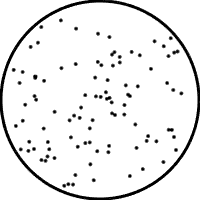Last week we modeled this process using a simple model that tracks D dots, counts how many of those D dots move in either direction, and then chooses the direction with the most number of dots.
We implemented this model with this code, which you hopefully recreated for yourself in last week's Assignment.
clear
% number of dots participants pay attention to
D = 100;
% viewing time in frames
T = 1;
% fraction of dots moving in the preferred direction
f = 0.6;
% initialize count variables
N_correct = 0;
N_wrong = 0;
% for loop to loop over all dots
for i = 1:D*T
% randomly sample the direction of this dot
dot_direction = binornd(1, f);
% update the counts
N_correct = N_correct + dot_direction;
N_wrong = N_wrong + (1 - dot_direction);
end
% display number of dots counted in "correct" direction
N_correct
% display number of dots counted in "wrong" direction
N_wrong
% compute accuracy of this choice
if N_correct > N_wrong
accuracy = 1
end
if N_correct < N_wrong
accuracy = 0
end
if N_correct == N_wrong
accuracy = binornd(1,0.5)
end
This is great, so far as it goes. But it's only one decision. If we want to simulate a whole experiment we need to be able to model multiple decisions. In addition, if we want to do anything with the resulting simulated data, we need to figure out a way to store the data too.

## The goal for this week

Our goal for this week is to build a model of multiple decisions in the dots task and use it to simulate a whole experiment. We will then analyze this "fake data" to produce a plot like this one from Gold and Shadlen in 2004. In their plot the data points are real data from actual monkeys performing the dots task. In our plot the data will come from the model.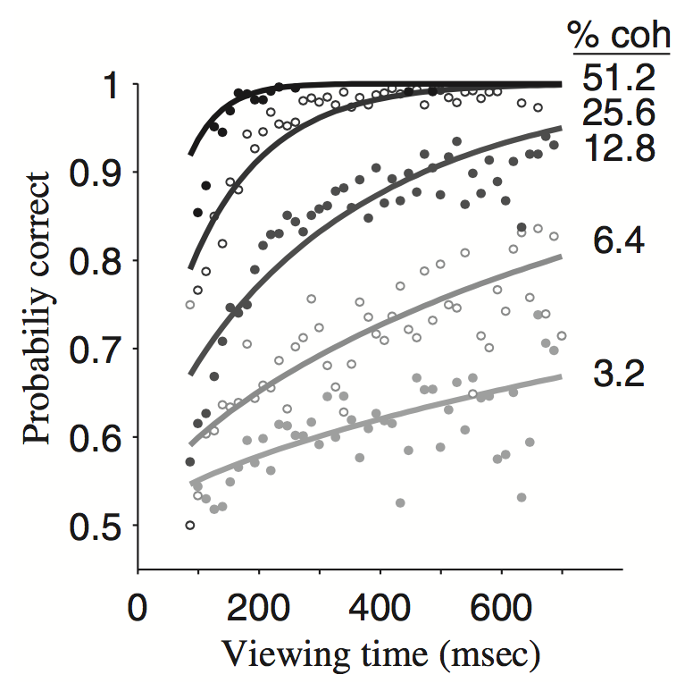## Changing the code to make more than one decision

We will make three changes to allow it to make multiple decisions:
1. Create a function that can implement one decision
2. Call this function inside a loop
3. Store the accuracy of each decision in a vector
4. Compute the average accuracy over these many decisions

### Make a function that implements one decision

The code we created last week works fine, but it's kind of cumbersome to use everytime we want to simulate a single decision. It would be much easier and cleaner for us if we could somehow encapsulate everything so that we can call all of that code with just a single line. Perhaps like this ...
accuracy = makeOneDecision(D, T, f);
Where makeOneDecision is the name of a function that takes variables D, T, and f as inputs.
Thankfully, Matlab allows us to do this relatively easily and if you can master this function functionality of Matlab it will make things much easier for you going forward in the class.
To create a function in Matlab we start by creating a new file (click on "New Script" on the toolbar or hit cmd+N or control+N when you're in the Editor window).
We then start the new file in a very specific way. The first thing we type has to be the word "function" followed by the way in which we want the command to be called. In our case this is
function accuracy = makeOneDecision(D, T, f)
Note we don't need the semi-colon at the end of the line.
After typing this line I always like to save the new file (by hitting Save on the EDITOR tab of the toolbar or cmd+S (mac) / control+S (windows)). This will pop up a window asking you where you want to save the file (put it into the Week_02 folder on your Dropbox).
*** IMPORTANT *** Make sure the name of your file matches the name of the function. Matlab does this automatically if you've input the "function" line, but this is a common source of bugs so watch out for it!
After that line, we need to copy in the code from last week for making one decision. Note, however, that we don't need the lines that define the variables D, T, and f, because those will be defined by how we call the function later on.
So our function code should look like this ...
function accuracy = makeOneDecision(D, T, f)
% initialize count variables
N_correct = 0;
N_wrong = 0;
% for loop to loop over all dots
for i = 1:D*T
% randomly sample the direction of this dot
dot_direction = binornd(1, f);
% update the counts
N_correct = N_correct + dot_direction;
N_wrong = N_wrong + (1 - dot_direction);
end
% compute accuracy of this choice
if N_correct > N_wrong
accuracy = 1;
end
if N_correct < N_wrong
accuracy = 0;
end
if N_correct == N_wrong
accuracy = binornd(1,0.5);
end
end
Note: I made a couple of small edits from the script code, can you see what they are and can you figure out why I made them?
And that's it ... we've made our function! You might be tempted at this point to run the function by hitting the run button (or hitting F5). If you do that you'll get an error message, the reason being that you haven't defined D, T and f yet. Don't worry - this is perfectly normal!
In general you won't be running functions using the run button. Instead you will be calling them from inside scripts (or even inside other functions). We'll do that now when we build a script to simulate multiple decisions ...
Now the function is defined, it's really easy to make multiple decisions. First, we create a new script. Then we clear and define our variables as before ...
clear
% number of dots participants pay attention to
D = 100;
% viewing time in frames
T = 1;
% fraction of dots moving in the preferred direction
f = 0.6;
Note that we also want to add a fourth variable "nDecisions" that tells us how many decisions we want to simulate
% number of decisions
nDecisions = 10;
Finally, we can actually simulate all those decisions using a for loop
for i = 1:nDecisions
accuracy = makeOneDecision(D, T, f);
end
This is great, but if you run this you might notice a problem in your Workspace ...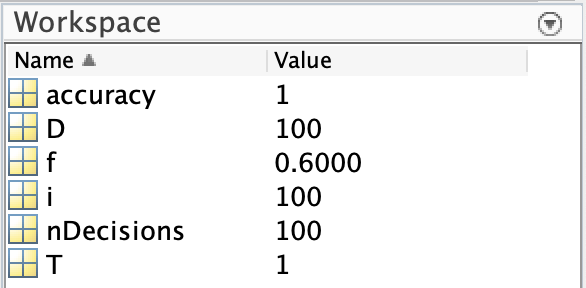Specifically the value of accuracy is just a single number (in my case 1). This is a problem because even though we simulated 100 decisions we only stored the accuracy of the last decision in the variable accuracy.
Instead what we would like to do is store the accuracies from every decision. To do that we are going to need vectors ...

### Storing accuracies with vectors

Roughly speaking, what we'd like to do when we store the accuracies is create something like a table, or spreadsheet where I have a list of entries telling me the accuracy on every trial.
A simple way to do this is with vectors. For our purposes, a vector is just a list of numbers and a simple way to create one for accuracies is like this ...
for i = 1:nDecisions
accuracy(i) = makeOneDecision(D, T, f);
end
where the only thing that's changed is the addition of "(i)" to the end of accuracy. What this parathetical i does is it indexes which element of the vector accuracy we wish to assign the output of makeOneDecision to.
On the first run through the loop, when i is 1, we index the first element of accuracy (accuracy(1)) and set its value to the output of the first decision.
On the second run through,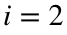, and we assign accuracy(2), and so on.
At the end we create a vector with nDecision numbers. This is relected in the Workspace like this ...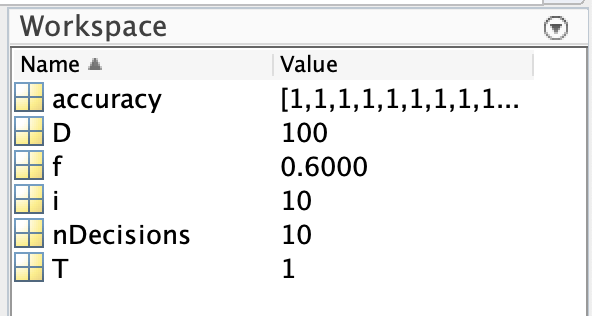where you can actually see the list of numbers (all 1s in my case).
Moreover, we can take a closer look at the vector by double clicking on it in the Workspace, which opens up a sort of spreadsheet view of the vector ...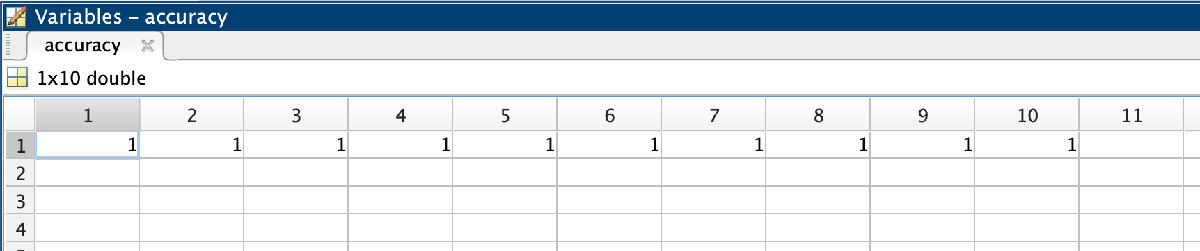Here you can see that the entries are all 1, i.e. the model is correct on every trial. This is because the dots decision is really easy if you have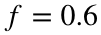and can track 100 dots.

## Taking the average of all the accuracies

Finally to get the average accuracy for this combination of parameters (D=100, f=0.6 and T=1) we need to take the mean of all of those accuracies. Of course, in the simulations above this is trivial (the mean is just 1), but in the general case it's not and in any case we'd like Matlab to do it automatically for us.
Thankfully Matlab provides a function to let us compute this easily. Intuitively it's called "mean" and it takes the mean of all the elements inside a vector. So to get the average accuracy we can write ...
AVERAGE_ACCURACY = mean(accuracy)

# Simulating multiple conditions

Great! We've simulated 10 decisions, with one set of parameters. Another way of saying this is we've simulated the model's behavior for one point on this plot (which corresponds to a particular configuration of f (which remember is similar to coherence) and T which is the viewing time.We're definitely making progress, but to really test our model we need to be able to simulate multiple conditions.
To do this, in the Assignment I'm going to ask you to make another function that simulates multiple decisions and outputs not the accuracy of one decision but the AVERAGE_ACCURACY of all decisions. This function you will then call like this ...
AVERAGE_ACCURACY = makeManyDecisions(D, T, f, nDecisions);
Assuming you manage to do that, let's use this makeManyDecisions function to make a version of the plot above ...
To simulate multiple conditions we will use a mixture of vectors, for loops and a new entity called a matrix.

## Vectors to define the possible values of T and f

To make the equivalent of the above plot for our model, we need to vary the viewing time T and the fraction of dots moving in the preferred direction f. To define the particular set of T and f values we want to use, we will create two vectors, one for the set of T values and the other for the set of f values.
For T, one way to define the list of values is like this ...
T_vals = [1 2 4 8];
where the square brackets [ and ] tells Matlab that we are creating a vector and the individual entries to the vector are separated by spaces. Here we have four entries corresponding to 1, 2, 4 and 8 frames of the movie.
This kind of manual definition for a vector works well when you only want a few values of T, but gets tedious if we want a lot of them. Another way to define a list like this is a particular use of the colon ":" key ... Specifically, if we'd like to set T_vals to be every whole number between 1 and 100 we could write
T_vals = [1:100];
This creates a vector with 100 elements which are all the numbers from 1 to 100. If you want to check for yourself paste this line into the Command Window, hit enter and check the vector for yourself in the Workspace.
Note: We've used this colon notation before when we defined the for loop. It means the same there as it does here - start from the first number (in this case 1) and go in steps of 1 to the last number (in this case 100).
But maybe we think that one hundred values for T is a bit much. Suppose we want the number to range between 1 and 100, but we only use a subset of the numbers (but still too many to enter manually).
One way of doing this is to use two colons, like this
T_vals = [1:20:100];
This will create a list of numbers between 1 and 100 in steps of 20. So this is equivalent to writing ...
T_vals = [1 21 41 61 81];
Note that 100 is not included in this list. If you want to get 100 in too, we can combine the colon notation with a space and then the 100 like this
T_vals = [1:20:100 100];
which is equivalent to
T_vals = [1 21 41 61 81 100];
For the actual simulation I suggest starting with
T_vals = [1:5:21];
If we assume that the dots stimulus is presented at 30 frames per second, then 21 frames corresponds to 700ms, which was the duration of the longest stimulus used in the monkey experiment.
For f we can use values that correspond to the coherences listed in the plot. First, let's create a vector of the coherences ...
COH = [3.2 6.4 12.8 25.6 51.2]/100;
where the "/ 100" part divides every number in the vector by 100 to make it a decimal rather than a percentage.
Then we can convert all of these coherences into f values using the equationwhich is implemented in Matlab as
f_vals = (COH / 100 + 1) / 2;

## for loops to go through all the values of T and f

Next we need to go through all the T and f values and compute the average accuracy of the model for each of them. To do this we need to use two for loops, one to loop through T_vals and the other to loop through f_vals. The Matlab notation for this is
for i = 1:length(T_vals)
for j = 1:length(f_vals)
AVERAGE_ACCURACY = makeManyDecisions(D, T_vals(i), f_vals(j), nDecisions);
end
end
There's quite a few new things here. First, is the "length" function. This is a super useful function that tells you the length (i.e. the number of elements in) your vector. So
length(T_vals)
returns the number of elements in T_vals (in our case 5). So the loop over i goes from 1 to 5.
Next, to loop through the f_vals, we used a different variable, j. Note that we can't use i again because we're already using that to loop over all the elements in T_vals.
Finally, to pull out a single element (i.e. a single value) from our vectors we refer to that element using parentheses
T_vals(i)
and
f_vals(j)
Running this code computes the average accuracy for each every combination of T and f in our T_vals and f_vals lists. It does not, however, store the results. For that we need matrices ...

## A matrix to store the results

Finally we need to store the results in a matrix. This is like a generalization of a vector to two dimensions. If a vector is a bunch of numbers arranged in a line, then a matrix is a bunch of numbers arranged in a table. To make a matrix in our code we just need to add indices to AVERAGE_ACCURACY, like this ...
for i = 1:length(T_vals)
for j = 1:length(f_vals)
AVERAGE_ACCURACY(i,j) = makeManyDecisions(D, T_vals(i), f_vals(j), nDecisions);
end
end
where the (i, j) refers to the "coordinates" of the element in the matrix.

## Putting it all together

So now our code for implementing simulating behavior in the task looks like this ...
Note: I've changed D to be 10 and nDecisions to be 1000. Setting D = 10 gets us close to the behavior of the monkeys. Setting nDecisions to 1000 gives us lots of data points to average over making our resulting curves relatively smooth.
Note also: When you run this on your machine you may want to reduce nDecisions to around 100. Even then, it may take a while to run on older / slower computers.
clear
% number of dots participants pay attention to
D = 10;
% viewing time in frames
T_vals = [1:5:21];
% fraction of dots moving in the preferred direction
COH = [3.2 6.4 12.8 25.6 51.2]/100;
f_vals = (COH + 1) / 2;
% number of decisions
nDecisions = 1000;
for i = 1:length(T_vals)
for j = 1:length(f_vals)
AVERAGE_ACCURACY(i,j) = makeManyDecisions(D, T_vals(i), f_vals(j), nDecisions);
end
end
Displaying the matrix gives us something like this, which you can also see if you click on AVERAGE_ACCURACY in the Workspace
AVERAGE_ACCURACY
AVERAGE_ACCURACY = 5×5
0.5615 0.5845 0.6580 0.7900 0.9665 0.6035 0.6740 0.8280 0.9770 1.0000 0.6335 0.7495 0.9175 0.9945 1.0000 0.6660 0.7830 0.9485 0.9995 1.0000 0.7000 0.8160 0.9730 1.0000 1.0000
Each column corresponds to a different value of f and each row to a different value of T. Going down the columns, as f increases the accuracy increases. Going across the rows, as T increases the accuracy increases. Both of these results make sense - the more dots moving in the preferred direction and the longer you view the stimulus for, the better your decision.

## Plotting the results

Finally, we need to plot the results. We can do this using the plot command.
plot(AVERAGE_ACCURACY)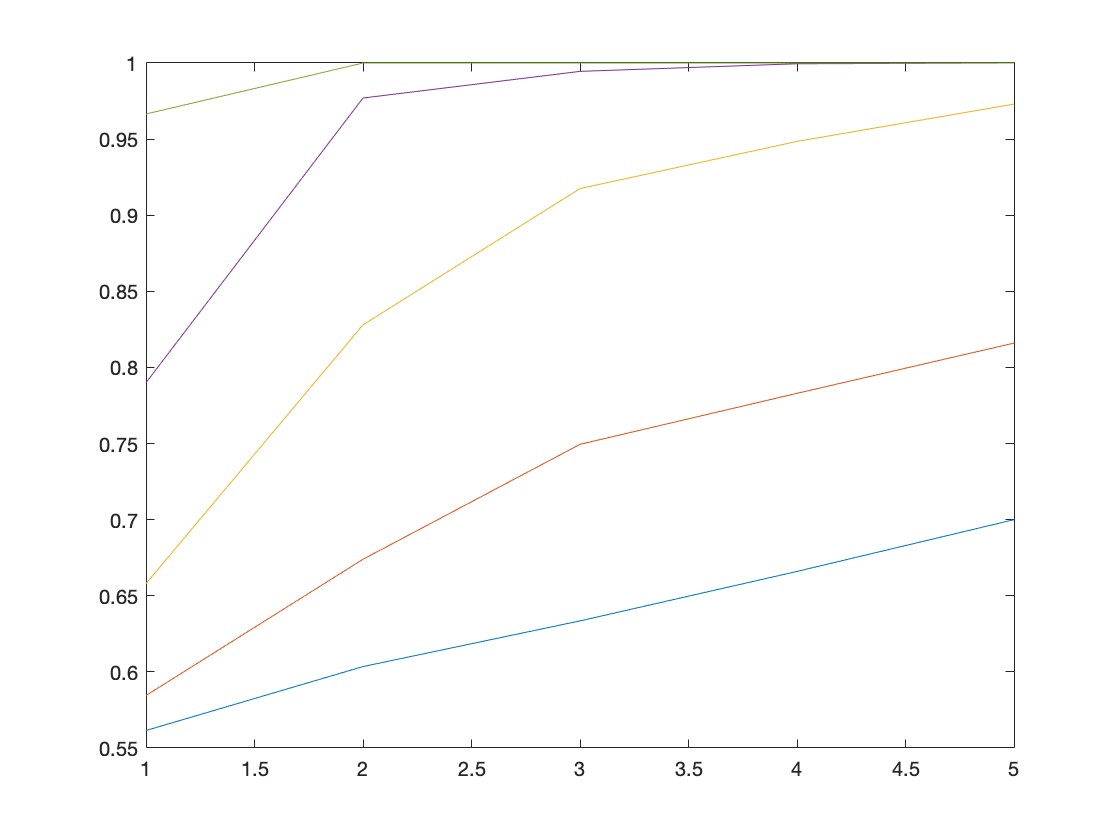Each line is a different column of the matrix and a different value of f. The x-axis corresponds to the index of T not its value. The y-axis is the average accuracy. Comparing this with the plot from the experiment suggests this model might be doing a pretty good job of capturing the behavior. But before we compare things in more depth, it would be nice to improve how this figure looks. Specifically it would be nice to
• put the value of T (not the index of T_vals) on the x-axis
• change the appearance of the lines
• label the axes
• put in a legend to label the lines
• increase the fontsize

### Put T on the x-axis

We can easily get the actual time on x-axis we can do this easily by slightly modifying the plot command
plot(T_vals*1000/30, AVERAGE_ACCURACY)